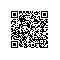# Java正则表达式

正则表达式通常处理方式：

1. Pattern p = Pattern.compile("a*b");
2.         Matcher m = p.matcher("aaaaab");
3.         boolean b = m.matches();

或者：System.out.println("abc".matches("..."));//String类中有match()方法

1. 构造 匹配
2.
3. 字符
4. x 字符 x
5. \\ 反斜线字符
6. \0n 带有八进制值 0 的字符 n (0 <= n <= 7)
7. \0nn 带有八进制值 0 的字符 nn (0 <= n <= 7)
8. \0mnn 带有八进制值 0 的字符 mnn（0 <= m <= 3、0 <= n <= 7）
9. \xhh 带有十六进制值 0x 的字符 hh
10. \uhhhh 带有十六进制值 0x 的字符 hhhh
11. \t 制表符 ('\u0009')
12. \n 新行（换行）符 ('\u000A')
13. \r 回车符 ('\u000D')
14. \f 换页符 ('\u000C')
15. \a 报警 (bell) 符 ('\u0007')
16. \e 转义符 ('\u001B')
17. \cx 对应于 x 的控制符
18.
19. 字符类
20. [abc] a、b 或 c（简单类） 【注意】非"abc"，而是"[abc]"
21. [^abc] 任何字符，除了 a、b 或 c（否定）
22. [a-zA-Z] a 到 z 或 A 到 Z，两头的字母包括在内（范围）
23. [a-d[m-p]] a 到 d 或 m 到 p：[a-dm-p]（并集）
24. [a-z&&[def]] d、e 或 f（交集）
25. [a-z&&[^bc]] a 到 z，除了 b 和 c：[ad-z]（减去）
26. [a-z&&[^m-p]] a 到 z，而非 m 到 p：[a-lq-z]（减去）
27.
28. 预定义字符类
29. . 任何字符（与行结束符可能匹配也可能不匹配）
30. \d 数字：[0-9]
31. \D 非数字： [^0-9]
32. \s 空白字符：[ \t\n\x0B\f\r]
33. \S 非空白字符：[^\s]
34. \w 单词字符：[a-zA-Z_0-9]
35. \W 非单词字符：[^\w]
36.
37. POSIX 字符类（仅 US-ASCII）
38. \p{Lower} 小写字母字符：[a-z]
39. \p{Upper} 大写字母字符：[A-Z]
40. \p{ASCII} 所有 ASCII：[\x00-\x7F]
41. \p{Alpha} 字母字符：[\p{Lower}\p{Upper}]
42. \p{Digit} 十进制数字：[0-9]
43. \p{Alnum} 字母数字字符：[\p{Alpha}\p{Digit}]
44. \p{Punct} 标点符号：!"#$%&'()*+,-./:;<=>?@[\]^_`{|}~ 45. \p{Graph} 可见字符：[\p{Alnum}\p{Punct}] 46. \p{Print} 可打印字符：[\p{Graph}\x20] 47. \p{Blank} 空格或制表符：[ \t] 48. \p{Cntrl} 控制字符：[\x00-\x1F\x7F] 49. \p{XDigit} 十六进制数字：[0-9a-fA-F] 50. \p{Space} 空白字符：[ \t\n\x0B\f\r] 51. 52. java.lang.Character 类（简单的 java 字符类型） 53. \p{javaLowerCase} 等效于 java.lang.Character.isLowerCase() 54. \p{javaUpperCase} 等效于 java.lang.Character.isUpperCase() 55. \p{javaWhitespace} 等效于 java.lang.Character.isWhitespace() 56. \p{javaMirrored} 等效于 java.lang.Character.isMirrored() 57. 58. Unicode 块和类别的类 59. \p{InGreek} Greek 块（简单块）中的字符 60. \p{Lu} 大写字母（简单类别） 61. \p{Sc} 货币符号 62. \P{InGreek} 所有字符，Greek 块中的除外（否定） 63. [\p{L}&&[^\p{Lu}]] 所有字母，大写字母除外（减去） 64. 65. 边界匹配器 66. ^ 行的开头 67.$ 行的结尾
68. \b 单词边界
69. \B 非单词边界
70. \A 输入的开头
71. \G 上一个匹配的结尾
72. \Z 输入的结尾，仅用于最后的结束符（如果有的话）
73. \z 输入的结尾
74.
75. Greedy 数量词
76. X? X，一次或一次也没有
77. X* X，零次或多次
78. X+ X，一次或多次
79. X{n} X，恰好 n 次
80. X{n,} X，至少 n 次
81. X{n,m} X，至少 n 次，但是不超过 m 次
82.
83. Reluctant 数量词
84. X?? X，一次或一次也没有
85. X*? X，零次或多次
86. X+? X，一次或多次
87. X{n}? X，恰好 n 次
88. X{n,}? X，至少 n 次
89. X{n,m}? X，至少 n 次，但是不超过 m 次
90.
91. Possessive 数量词
92. X?+ X，一次或一次也没有
93. X*+ X，零次或多次
94. X++ X，一次或多次
95. X{n}+ X，恰好 n 次
96. X{n,}+ X，至少 n 次
97. X{n,m}+ X，至少 n 次，但是不超过 m 次
98.
99. Logical 运算符
100. XY X 后跟 Y
101. X|Y X 或 Y
102. (X) X，作为捕获组
103.
104. Back 引用
105. \n 任何匹配的 nth 捕获组
106.
107. 引用
108. \ Nothing，但是引用以下字符
109. \Q Nothing，但是引用所有字符，直到 \E
110. \E Nothing，但是结束从 \Q 开始的引用
111.
112. 特殊构造（非捕获）
113. (?:X) X，作为非捕获组
114. (?idmsux-idmsux)  Nothing，但是将匹配标志i d m s u x on - off
115. (?idmsux-idmsux:X)   X，作为带有给定标志 i d m s u x on - off
118. (?<=X) X，通过零宽度的正 lookbehind
119. (?<!X) X，通过零宽度的负 lookbehind
120. (?>X) X，作为独立的非捕获组

场景1：将所有数字用'-'替换

1. String s = "abc123def456hijas2kj23jl1"
2.     String dest = s.replaceAll("\\d""-");
3.     System.out.println(dest);

场景2：判断是否是电子邮箱

1. String s = "tianya@sina.com"
2.         System.out.println(s.matches(".+@.+\\..+"));

【注】\\.代表'.',表示真正的‘点’号，否则会代表‘任意字符’

场景3：

1. String email = "qing.qingbyqing@gmail.vip.com";//邮箱测试
2.        FormValid.isValidEmail(email);
3.         String strIp="1.10.111.255";//IP地址测试
4.        FormValid.isValidIp(strIp);
5.
6.         String sDate="03/03/1911";
7.         FormValid.isValidDate(sDate);//日期测试
8.
9.
10.       String strNum = "4432123456788881";
11.       w(FormValid.isValidCard(strNum));//信用卡测试

本文转自 tianya23 51CTO博客，原文链接：http://blog.51cto.com/tianya23/673756，如需转载请自行联系原作者使用钉钉扫一扫加入圈子
+ 订阅